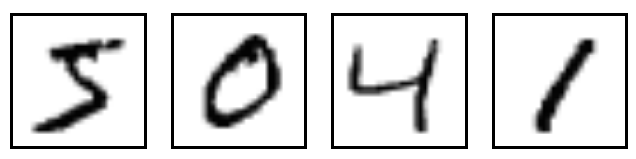Tag - Handwritten Recognition

## 什么是 MNIST

MNIST 是深度学习巨巨 Yann LeCun 维护的一套手写数字图像数据库，包含 60000 个训练样本和 10000 个测试样本，所有这些图像已经做过规范化及居中处理，拥有同样的固定尺寸，使用起来非常方便。下面是其中几个例子。## Let's do it, but how?

### 读取 MNIST 数据

```from tensorflow.examples.tutorials.mnist import input_data
```

`input_data` 封装了 MNIST 数据的下载、解析功能，`read_data_sets()` 返回三部分数据：55000 个训练样本 `mnist.train`，5000 个验证样本 `mnist.validation`（注意到没有，这 5000 是从原始的 60000 个训练样本上拿出来的），10000 个测试样本 `mnist.test`

### 建立 DNN 模型

```import tensorflow as tf

X = tf.placeholder(tf.float32, [None, 784])
Y = tf.placeholder(tf.float32, [None, 10])
p_keep_input = tf.placeholder(tf.float32)
p_keep_hidden = tf.placeholder(tf.float32)

def init_weights(shape):
return tf.Variable(tf.random_normal(shape, stddev=0.01))

w_h = init_weights([784, 1024])
w_h2 = init_weights([1024, 625])
w_o = init_weights([625, 10])

def model(X, w_h, w_h2, w_o, p_keep_input, p_keep_hidden):
X = tf.nn.dropout(X, p_keep_input)

h = tf.nn.relu(tf.matmul(X, w_h))
h = tf.nn.dropout(h, p_keep_hidden)

h2 = tf.nn.relu(tf.matmul(h, w_h2))
h2 = tf.nn.dropout(h2, p_keep_hidden)

return tf.matmul(h2, w_o)

py_x = model(X, w_h, w_h2, w_o, p_keep_input, p_keep_hidden)
```

### 训练模型

```cost = tf.reduce_mean(tf.nn.softmax_cross_entropy_with_logits(logits=py_x, labels=Y))
train_op = tf.train.RMSPropOptimizer(0.001, 0.9).minimize(cost)
```

### 评估模型

```predict_acc = tf.reduce_mean(tf.cast(tf.equal(tf.argmax(py_x, 1), tf.argmax(Y, 1)), tf.float32))
```

```epoch_count = 20000
batch_size = 50
keep_input = 0.8
keep_hidden = 0.75

with tf.Session() as sess:
sess.run(tf.global_variables_initializer())

step = 0
for i in xrange(epoch_count):
step += 1
batch_x, batch_y = mnist.train.next_batch(batch_size)
sess.run(train_op, feed_dict={X: batch_x, Y: batch_y, p_keep_input: keep_input, p_keep_hidden: keep_hidden})
if step % 100 == 0:
loss, acc = sess.run([cost, predict_acc], feed_dict={X: batch_x, Y: batch_y, p_keep_input: 1., p_keep_hidden: 1.})
print("Epoch: {}".format(step), "\tLoss: {:.6f}".format(loss), "\tTraining Accuracy: {:.5f}".format(acc))
print("Testing Accuracy: {:0.5f}".format(sess.run(predict_acc, feed_dict={X: mnist.test.images, Y: mnist.test.labels, p_keep_input: 1., p_keep_hidden: 1.})))
```